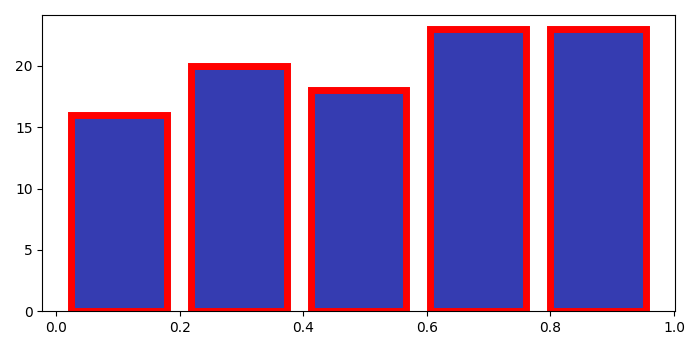# Plotting a transparent histogram with non-transparent edge in Matplotlib

To plot a transparent histogram with non-transparent edge, we can take the following steps−

• Create a set of random data points (y).
• Initialize the number of bins to be drawn.
• To plot the histogram, we can use hist() method with edge color and facecolor tuples
• To display the figure, use show() method.

## Example

import numpy as np
from matplotlib import pyplot as plt
plt.rcParams["figure.figsize"] = [7.00, 3.50]
plt.rcParams["figure.autolayout"] = True
y = np.random.rand(100)
nbins = 5
plt.hist(y, bins=nbins, edgecolor=(1, 0, 0, 1), lw=5, facecolor=(.09, .12, .65, .87), rwidth=0.8)
plt.show()

## Output